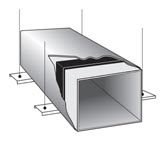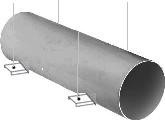English    中文

# Air flow unit online conversion tool

Category:

Rectangle DuctCircular Ductft/min
(LFM)
m/s
miles/hr (MPH)
ft3/min (CFM)
m3/hr
L/s

### App Description

Air flow rate unit conversion

The speed of the air is measured at a specific location, with respect to the measurement of the air or gas displacement rate.

Air velocity (distance per unit time) is usually expressed in feet per minute (LFM). By multiplying the air flow rate by the cross-sectional area of ​​the pipe, you can determine the amount of air flowing through a point in the pipe per unit time. Volumetric flow is usually expressed in cubic feet per minute (CFM).

The concept of air flow rate is applied to daily air conditioning, heating and ventilation.

Enter the air flow rate value, select a rectangular tube or a round tube, and enter the size of the corresponding tube. Click “Calculate” and the result will be displayed.

### Usage example

Input data: air flow rate is 100LFM (feet per minute), select the rectangular tube, click the small box above the rectangular tube, check the checkmark, and then enter the height (H) and width (W) of the rectangular tube, each input 2 , click "Calculate" to display the result.

Output: Air flow rate units were converted to 100 ft/min; 0.51 m/sec; 1.1 mph/2.78 cubic feet/minute; 4.72 cubic meters/hour; 1.31 liters/second.

##### for comments!

Comment list ( 0 )

Evaluation
App classification
Recent used